# Reinforcement Learning II: A2C

In this tutorial we will train a reinforcement learning agent to play Blood Bowl using the synchronous advantage actor-critic (A2C) algorithm, which is a simpler variant of A3C (Asynchronous Advantage Actor-Critic). This tutorial will be hard to follow without a basic understanding of deep reinforcement learning, including concepts such as neural networks, convolution, back-propagation, gradient descent, temporal difference learning, and parallelization in RL. If you are unfamiliar with some of these concepts, we recommend that you first read through some of these resources:

The code presented in this tutorial is based on examples/a2c/a2c_example.py which is inspired by a pytorch implementation of A2C by Pratyush Kar and the botbowl-A2C repository by Lasse Møller Uth and Christopher Jakobsen, which is no longer compatible with the newer versions of botbowl.

In this tutorial, we focus on three small variants of Blood Bowl:

• botbowl-1-v2: 1 player on a 4x3 pitch for each team.
• botbowl-3-v2: 3 player on a 12x5 pitch for each team.
• botbowl-5-v2: 5 player on a 16x9 pitch for each team.

It was previously shown in the paper Blood Bowl: A New Board Game Challenge and Competition for AI that A2C with reward shaping was able to learn strong policies in these variants against a random opponent.

In future tutorials, we will attempt to:

1. scale this approach to the 11-player variant of the game
2. apply self-play to achieve a robust policy against diverse opponents

The v2 environments don’t use pathfinding, i.e. you can only move one square at each step. The results shown in the first two RL tutorials use v2. The v3 environments (simply change the name from botbowl-1-v2 to botbowl-1-v3) does use pathfinding such that players can move several squares in one action. The games thus have fewer steps in v3 but are slower.

## Observation and Action Space

We will use the default observation space in botbowl.

Action spaces can be split into branches, such that one action is sampled from each branch. In botbowl, we need to sample an action type, and then for some action types also a position. This can be achieved using three branches; 1) action type, 2) x-coordinate, and 3) y-coordinate. This approach was e.g. applied in preliminary results presented in StarCraft II: A New Challenge for Reinforcement Learning since StarCraft has a similar action space.

Instead of using branching, we believe it is more efficient to unroll the entire action space into one branch. In this approach, we have one action for each non-spatial action as well as W * H actions for each spatial action type, where W and H is the width and height of the spatial feature layers which are equal to the board size plus a one-tile padding, since push actions can be out of bounds.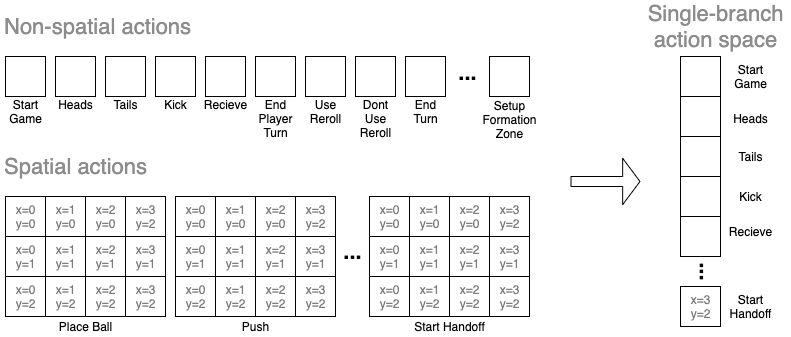We compute batches of action masks from the spatial feature layers that represent the available spatial actions. Action masks are computed as vectors of the same size as the one-branch action space as seen above:

``````def compute_action_masks(observations):
m = False
for ob in observations:
i = 0
for action_type in non_spatial_action_types:
i += 1
for action_type in spatial_action_types:
if ob['available-action-types'][action_type.name] == 0:
elif ob['available-action-types'][action_type.name] == 1:
position_mask = ob['board'][f"{action_type.name.replace('_', ' ').lower()} positions"]
for j in range(board_squares):
i += board_squares
if m:
``````

We use the followin function to convert back to the original action space.

``````def compute_action(action_idx):
if action_idx < len(non_spatial_action_types):
return non_spatial_action_types[action_idx], 0, 0
spatial_idx = action_idx - num_non_spatial_action_types
spatial_pos_idx = spatial_idx % board_squares
spatial_y = int(spatial_pos_idx / board_dim)
spatial_x = int(spatial_pos_idx % board_dim)
spatial_action_type_idx = int(spatial_idx / board_squares)
spatial_action_type = spatial_action_types[spatial_action_type_idx]
return spatial_action_type, spatial_x, spatial_y
``````

## Policy Network

We employ a relatively simple neural network architecture for the policy. The spatial feature layers are fed through two convolutional layers that use 3x3 filters with padding and stride 1. This maintains the size of the spatial input. The output of the this stream is flattened and then concatenated with the non-spatial input. The resulting vector then goes through a fully-connected layer and is then split up into the action output and state-value output. The action output is a fully-connected layer with a softmax activation function forming a distribution over actions. The state-value output is also fully-connected layer but without any activation function.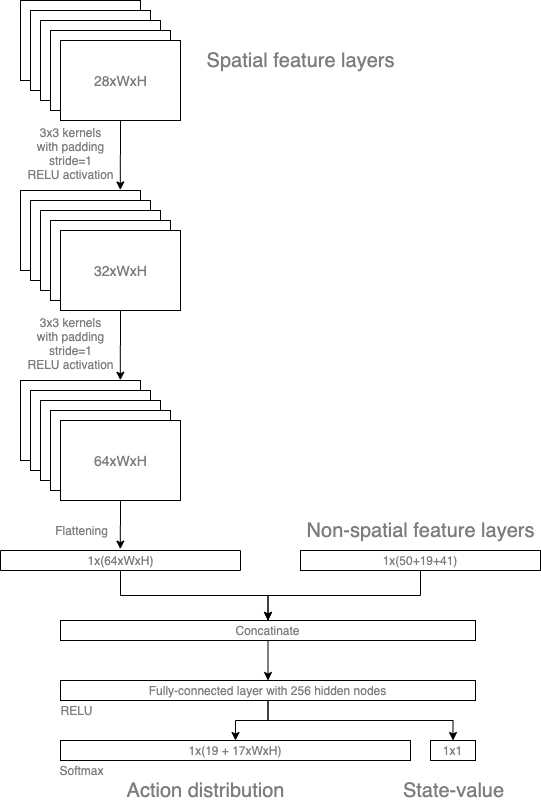We used pytorch to implement this policy network:

``````class CNNPolicy(nn.Module):
def __init__(self, spatial_shape, non_spatial_inputs, hidden_nodes, kernels, actions):
super(CNNPolicy, self).__init__()

# Spatial input stream
self.conv1 = nn.Conv2d(spatial_shape, out_channels=kernels, kernel_size=3, stride=1, padding=1)
self.conv2 = nn.Conv2d(in_channels=kernels, out_channels=kernels, kernel_size=3, stride=1, padding=1)

# Non-spatial input stream
self.linear0 = nn.Linear(non_spatial_inputs, hidden_nodes)

# Linear layers
stream_size = kernels * spatial_shape * spatial_shape
stream_size += hidden_nodes
self.linear1 = nn.Linear(stream_size, hidden_nodes)

# The outputs
self.critic = nn.Linear(hidden_nodes, 1)
self.actor = nn.Linear(hidden_nodes, actions)

self.train()
self.reset_parameters()

...

def forward(self, spatial_input, non_spatial_input):
"""
The forward functions defines how the data flows through the graph (layers)
"""
# Spatial input through two convolutional layers
x1 = self.conv1(spatial_input)
x1 = F.relu(x1)
x1 = self.conv2(x1)
x1 = F.relu(x1)

# Concatenate the input streams
flatten_x1 = x1.flatten(start_dim=1)

x2 = self.linear0(non_spatial_input)
x2 = F.relu(x2)

flatten_x2 = x2.flatten(start_dim=1)
concatenated = torch.cat((flatten_x1, flatten_x2), dim=1)

# Fully-connected layers
x3 = self.linear1(concatenated)
x3 = F.relu(x3)

# Output streams
value = self.critic(x3)
actor = self.actor(x3)

# return value, policy
return value, actor
``````

To sample an action from the action output we use the following two functions.

``````class CNNPolicy(nn.Module):
...
actions = action_probs.multinomial(1)  # Sample actions
return values, actions

values, actions = self(spatial_input, non_spatial_input)
action_probs = F.softmax(actions, dim=1)
return values, action_probs
``````

Notice that an action mask is used, i.e. we only sample for actions that are actually available. We do this by overriding the action output of all unavailable actions, setting them to the value `float('-inf')` since softmax will compute such values to 0. Notice, that these functions are called with batches of observations and thus batches of actions are returned.

## Reward Shaping

The default rewards in botbowl are based on the game outcome. To ease the learning, we will shape the reward function in several ways. First, we define the reward value of certain events. E.g. if the agent scores a touchdown it is rewarded with a value of 1 and if the opponent scores it is rewarded (punished) with a value of -1.

``````# --- Reward function ---
# You need to improve the reward function before it can learn anything on larger boards.
# Outcomes achieved by the agent
rewards_own = {
OutcomeType.TOUCHDOWN: 1,
OutcomeType.SUCCESSFUL_CATCH: 0.1,
OutcomeType.INTERCEPTION: 0.2,
OutcomeType.SUCCESSFUL_PICKUP: 0.1,
OutcomeType.FUMBLE: -0.1,
OutcomeType.KNOCKED_DOWN: -0.1,
OutcomeType.KNOCKED_OUT: -0.2,
OutcomeType.CASUALTY: -0.5
}
rewards_opp = {
OutcomeType.TOUCHDOWN: -1,
OutcomeType.SUCCESSFUL_CATCH: -0.1,
OutcomeType.INTERCEPTION: -0.2,
OutcomeType.SUCCESSFUL_PICKUP: -0.1,
OutcomeType.FUMBLE: 0.1,
OutcomeType.KNOCKED_DOWN: 0.1,
OutcomeType.KNOCKED_OUT: 0.2,
OutcomeType.CASUALTY: 0.5
}
# Outcomes achieved by the opponent
ball_progression_reward = 0.005
``````

Additionally, we give the agent a reward for moving the ball toward the opponent endzone. Here, we give a reward of 0.005 for every square the ball is moved closer. The reward function itself is defined like this:

``````def reward_function(env, info, shaped=False):
r = 0
for outcome in env.get_outcomes():
if not shaped and outcome.outcome_type != OutcomeType.TOUCHDOWN:
continue
team = None
if outcome.player is not None:
team = outcome.player.team
elif outcome.team is not None:
team = outcome.team
if team == env.own_team and outcome.outcome_type in rewards_own:
r += rewards_own[outcome.outcome_type]
if team == env.opp_team and outcome.outcome_type in rewards_opp:
r += rewards_opp[outcome.outcome_type]
if info['ball_progression'] > 0:
r += info['ball_progression'] * ball_progression_reward
return r
``````

`env.get_outcomes()` returns the outcomes since last step.

## Parallelization

A2C uses parallel worker processes to speed up the collection of experiences. First, we define a worker function that we will run in a new process:

``````def worker(remote, parent_remote, env, worker_id):
parent_remote.close()

steps = 0
tds = 0

while True:
command, data = remote.recv()
if command == 'step':
steps += 1
action = data
obs, reward, done, info = env.step(action)
tds_scored = info['touchdowns'] - tds
tds = info['touchdowns']
reward_shaped = reward_function(env, info, shaped=True)
if done or steps >= reset_steps:
# If we  get stuck or something - reset the environment
if steps >= reset_steps:
print("Max. number of steps exceeded! Consider increasing the number.")
done = True
obs = env.reset()
steps = 0
tds = 0
remote.send((obs, reward, reward_shaped, tds_scored, done, info))
elif command == 'reset':
steps = 0
tds = 0
obs = env.reset()
remote.send(obs)
elif command == 'render':
env.render()
elif command == 'close':
break
``````

Notice the `while` loop that will run until it is terminated (when a ‘close’ command is called) and will execute steps in the environment when queried. We use a meta-environment class called `VecEnv` that abstracts the communication with these workers by acting as list of botbowl environments. We can thus call `step()` with a list of actions, one for each environment, and it returns a list of future observations and rewards. This is practical as we can query our policy network with a batch of observations.

``````class VecEnv():
def __init__(self, envs):
"""
envs: list of botbowl environments to run in subprocesses
"""
self.closed = False
nenvs = len(envs)
self.remotes, self.work_remotes = zip(*[Pipe() for _ in range(nenvs)])

self.ps = [Process(target=worker, args=(work_remote, remote, env, envs.index(env)))
for (work_remote, remote, env) in zip(self.work_remotes, self.remotes, envs)]

for p in self.ps:
p.daemon = True  # If the main process crashes, we should not cause things to hang
p.start()
for remote in self.work_remotes:
remote.close()

def step(self, actions):
cumul_rewards = None
cumul_shaped_rewards = None
cumul_tds_scored = None
cumul_dones = None
for remote, action in zip(self.remotes, actions):
remote.send(('step', action))
results = [remote.recv() for remote in self.remotes]
obs, rews, rews_shaped, tds, dones, infos = zip(*results)
if cumul_rewards is None:
cumul_rewards = np.stack(rews)
cumul_shaped_rewards = np.stack(rews_shaped)
cumul_tds_scored = np.stack(tds)
else:
cumul_rewards += np.stack(rews)
cumul_shaped_rewards += np.stack(rews_shaped)
cumul_tds_scored += np.stack(tds)
if cumul_dones is None:
cumul_dones = np.stack(dones)
else:
cumul_dones |= np.stack(dones)
return np.stack(obs), cumul_rewards, cumul_shaped_rewards, cumul_tds_scored, cumul_dones, infos

def reset(self):
for remote in self.remotes:
remote.send(('reset', None))
return np.stack([remote.recv() for remote in self.remotes])

def render(self):
for remote in self.remotes:
remote.send(('render', None))
return

def close(self):
if self.closed:
return

for remote in self.remotes:
remote.send(('close', None))
for p in self.ps:
p.join()
self.closed = True

@property
def num_envs(self):
return len(self.remotes)
``````

## A2C Configurations

We use the following configurations:

``````# Training configuration
num_steps = 10000000
num_processes = 8
steps_per_update = 20  # We take 20 steps in every environment before updating
learning_rate = 0.001
gamma = 0.99
entropy_coef = 0.01
value_loss_coef = 0.5
log_interval = 50
save_interval = 500

# Environment
env_name = "botbowl-1-v2"
# env_name = "botbowl-3-v2"
# env_name = "botbowl-5-v2"
# env_name = "botbowl-7-v2"
# env_name = "botbowl-v2"

reset_steps = 2000  # The environment is reset after this many steps it gets stuck

# If set to False, the agent will only play as the home team and you would have to flip the state to play both sides.
botbowlEnv.play_on_both_sides = False

# Architecture
num_hidden_nodes = 256
num_cnn_kernels = [32, 64]

model_name = env_name
log_filename = "logs/" + model_name + ".dat"
``````

If `botbowlEnv.play_on_both_sides = False`, then the agent will only play as the home team. If set to `True`, it will also play as the away team and thus has to deal with flipped observations. We set this to False for now. We believe it is easier, in the future, to flip the observations data when playing as the away team rather learning how to play with on both sides.`

If for some reason the game halts or the agent is unable to execute a valid action, the game is reset after 2000 steps (`reset_steps`).

## Training

We make the code agnostic to which variant of botbowl we are using by first computing the size of action and observation space.

``````def make_env(worker_id):
print("Initializing worker", worker_id, "...")
env = gym.make(env_name)
return env

es = [make_env(i) for i in range(num_processes)]
envs = VecEnv([es[i] for i in range(num_processes)])

spatial_obs_space = es.observation_space.spaces['board'].shape
board_dim = (spatial_obs_space, spatial_obs_space)
board_squares = spatial_obs_space * spatial_obs_space

non_spatial_obs_space = es.observation_space.spaces['state'].shape + es.observation_space.spaces['procedures'].shape + es.observation_space.spaces['available-action-types'].shape
non_spatial_action_types = botbowlEnv.simple_action_types + botbowlEnv.defensive_formation_action_types + botbowlEnv.offensive_formation_action_types
num_non_spatial_action_types = len(non_spatial_action_types)
spatial_action_types = botbowlEnv.positional_action_types
num_spatial_action_types = len(spatial_action_types)
num_spatial_actions = num_spatial_action_types * spatial_obs_space * spatial_obs_space
action_space = num_non_spatial_action_types + num_spatial_actions
``````

We then instantiate our policy model using the computed sizes and an RMSprop optimizer. A memory store is then created that will contain trajectories of our agent. Trajectories will be 20 steps long for each worker.

``````# MODEL
ac_agent = CNNPolicy(spatial_obs_space, non_spatial_obs_space, hidden_nodes=num_hidden_nodes, kernels=num_cnn_kernels, actions=action_space)

# OPTIMIZER
optimizer = optim.RMSprop(ac_agent.parameters(), learning_rate)

# MEMORY STORE
memory = Memory(steps_per_update, num_processes, spatial_obs_space, (1, non_spatial_obs_space), action_space)

# Reset environments
obs = envs.reset()
spatial_obs, non_spatial_obs = update_obs(obs)

memory.spatial_obs.copy_(spatial_obs)
memory.non_spatial_obs.copy_(non_spatial_obs)
``````

When the memory is full (20 steps has been reached), reward values are discounted and training is performed on the data in the memory where after it is flushed.

Take a look for yourself at the remaining code in examples/a2c/a2c_example.py to understand how the learning works. Because the neural network is written in pytorch, you can run the code in debug mode and go through the code line by line.

## Results

The training process is plotted as the script runs. Here are the results we got on the three small botbowl environments.

### botbowl-1-v2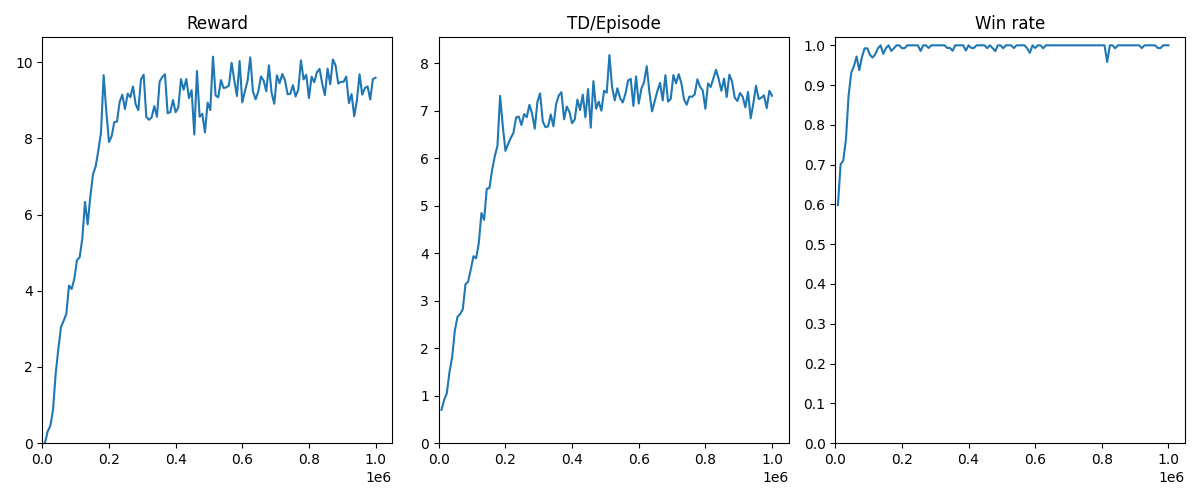### botbowl-3-v2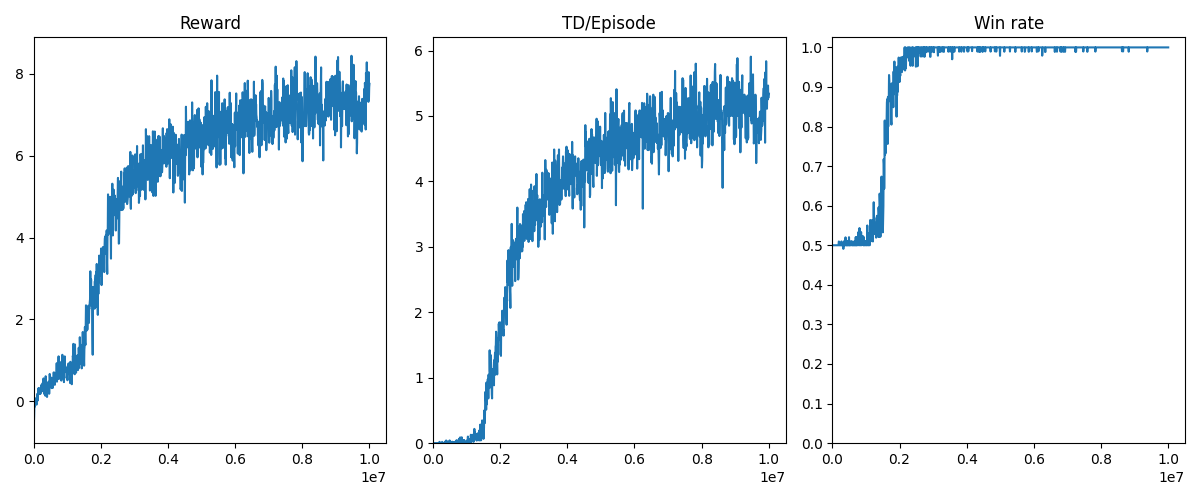### botbowl-5-v2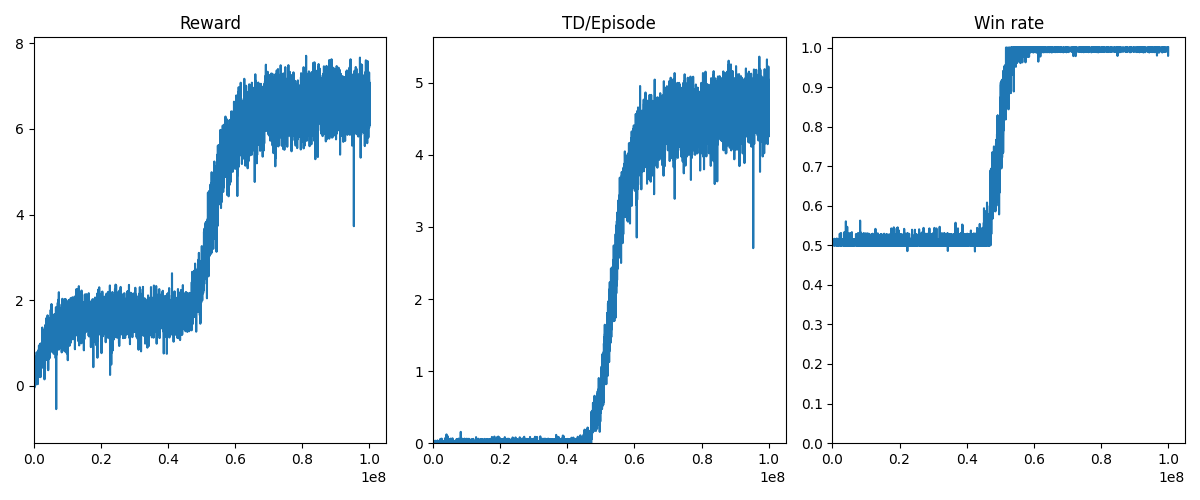Run the script to see if you can reproduce some of the results. Can you find better hyper-parameters or reward shaping rules?

## Next Steps

We were able to learn strong policies for the one, three, and five-player variants of Blood Bowl against a random opponent. In the next tutorial, we will improve our code to use several enhancements to reach the same results on the 11-player variant.